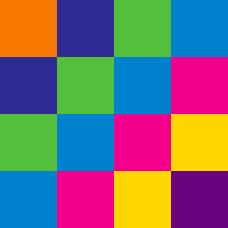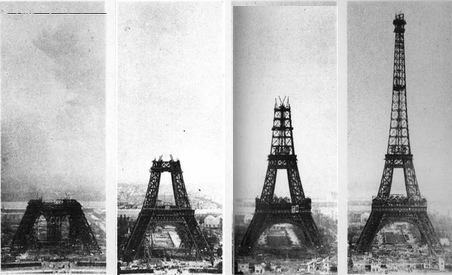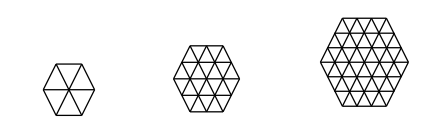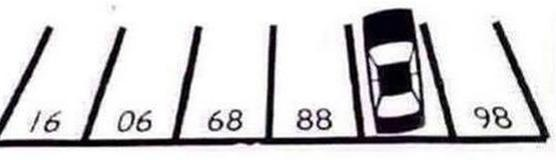Basic Mathematics

# Pattern Recognition: Level 2 ChallengesThe Eiffel Tower was the tallest structure in the world until 1930. If the first three images above were taken on March 1888, July 1888, and November 1888, which of the following dates is the most reasonable for the final picture of the completed tower?Following the pattern of the first 3 figures above, how many little triangles are there in the $9^\text{th}$ figure?

What is the next column?

$\begin{matrix} 35 & 42 & 49 \\ 40 & 48 & 56 \\ 45 & 54 & 63 \end{matrix}\quad\begin{matrix} ? \\ ? \\ ? \end{matrix}$

One day, when Fred was bored, he listed the integers from 1 to 9001 on the board. Let the digit that he wrote the most be $a$, and the digit that he wrote the least be $b$. Find $a+b$.What number is this car parked in?

×